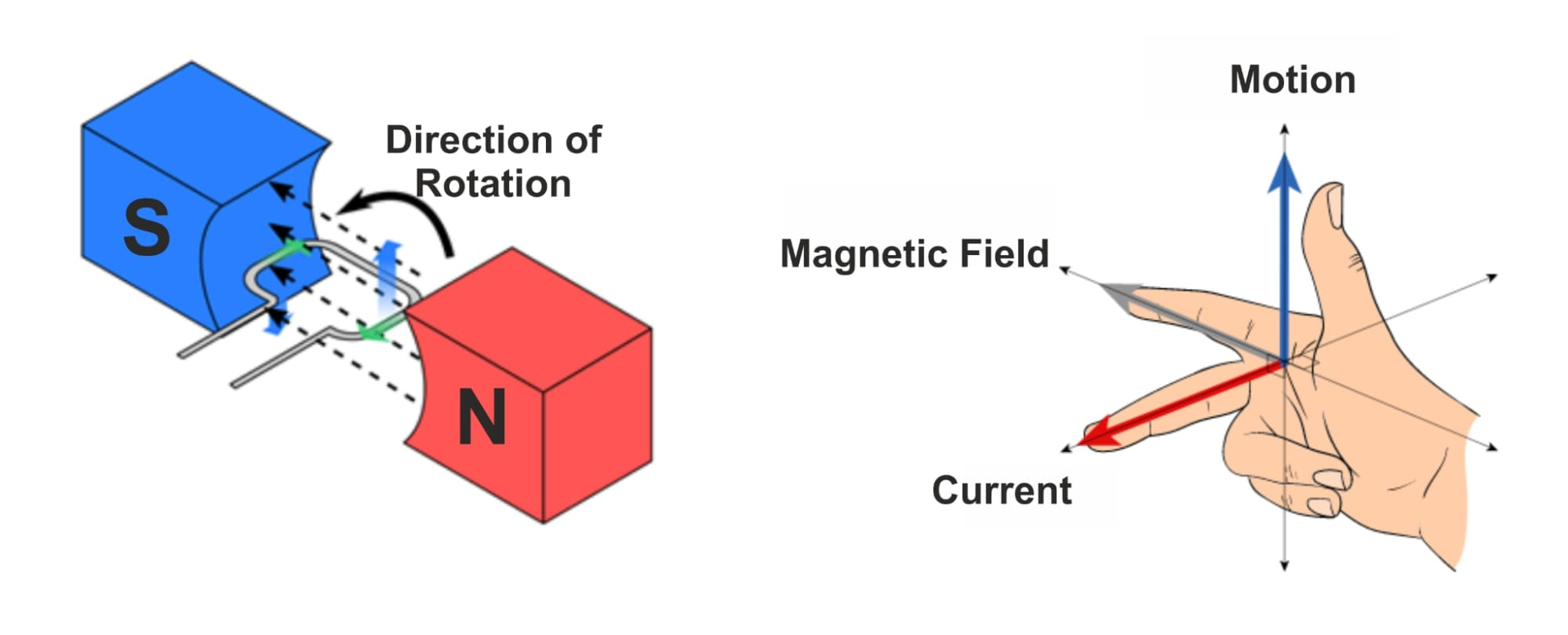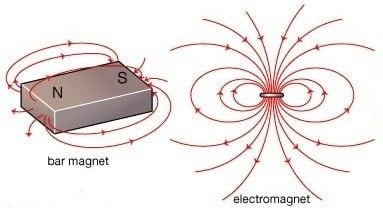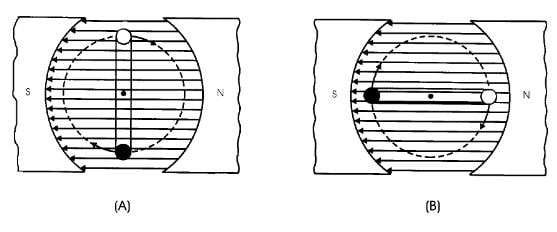Technical Article

# What is Electromagnetic Induction?

September 11, 2021 by Alex Roderick

## Electromagnetic induction refers to the production of an electromotive force (emf) across an electrical conductor that experiences a changing magnetic field.

Inductance (L) is the property of a device or circuit that causes it to oppose a change in current due to energy stored in a magnetic field. The current flowing in a coil produces a field that expands out of and surrounds the conductor. Energy is stored in that field. When the source voltage goes from peak to zero, the energy in the field is returned to the coil and converted back to electrical energy.

The three requirements for electromagnetic induction are a conductor, a magnetic field, and relative motion between the conductor and the field.

Note that the AC power flowing through a conductor generates an expanding and collapsing magnetic field.

The direction of the magnetic field around a conductor can be determined using the right-hand rule for conductors.

The polarity of current flow in the conductor is determined by the application of the right-hand rule for generators. See Figure 1. In the right-hand rule for generators, the index finger should be pointed in the direction of the magnetic field. The thumb points in the direction of motion of the conductor through the field, and the middle finger, which should be extended out at 90° from the index finger, will indicate the direction of current flow in the conductor.##### Figure 1. The polarity of current flow in the conductor is determined by the application of the right-hand rule for generators.

The amount of electromagnetic induction in a conductor is determined by the number of coil turns (loops), the strength of the magnetic field, relative motion speed, and angle of cutting motion and is given by the following formula:

$V=\frac{\phi \times S}{{{10}^{8}}}$

Where

$$V$$ = maximum induced voltage (in V)

$$\phi$$ = number of magnetic lines of force (in Mx)

$$S$$ = speed of relative motion (cycles per second)

Example: What is the maximum induced voltage when a conductor loop cuts 100,000,000 magnetic lines of force two times per second?

$V=\frac{\phi \times S}{{{10}^{8}}}=\frac{100,000,000\times 2}{{{10}^{8}}}=2\text{ }V$

## Number of Coil Turns

When the number of coil turns is increased, the level of induced voltage is also increased. For example, if 0.5 V were induced into one coil turn, then 2 V would be induced into four coil turns. The level of induced voltage is directly proportional to the number of coil turns. The number of coil turns is the multiplier when all other factors are held constant.

## Strength of Magnetic Field

When permanent magnets are employed as the source for magnetic lines of force to induce a voltage, the number of magnetic lines of force is constant. Electromagnets are typically used to adjust the number of magnetic lines of force (see Figure 2). Increasing resistance in a conductor results in lower current flow and fewer magnetic lines of force. Decreasing resistance in a conductor results in higher current flow and more magnetic lines of force. With all other factors held constant, increasing the magnetic lines of force results in a higher voltage being induced into the conductor. There must be relative motion between the magnetic lines of force and the conductor in order for a voltage to be induced. If a conductor is resting in the flux field, or if the conductor and the flux field are both moving together in the same direction with the same velocity, no voltage is induced.## Relative Motion

Relative motion is the speed at which a conductor cuts a magnetic field. When relative motion occurs between a field created by magnetic lines of force and a conductor, voltage is induced into the conductor. Increasing the speed at which the conductor cuts the magnetic field increases the induced voltage. The amount of induced voltage is directly proportional to the velocity of the cutting motion.

## Angle of Cutting Motion

The angle at which the conductor cuts the magnetic field affects the amount of induced voltage (see Figure 3).##### Figure 3. The angle at which the conductor cuts the magnetic field affects the amount of induced voltage.